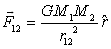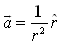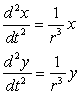## Newton's Law of Gravitation

One of the most studied central force problems is Newton's universal law of gravitation.  This law states that two spherical particles of mass M1 and M2 attracted each other with a force F12 that is inversely proportional to their separation squared and is directed along a line connecting their centersThe direction of the force on M1 is indicated by a unit vector "r-hat" pointing from M1 to M2. The proportionality constant G depends on the system of units and takes the value G = 6.67x10-11 m3/kg.s in the metric system.

Because two gravitationally interacting particles orbit in a plane about their common center of mass, we choose a coordinate system with the center of mass at the origin.  Intermediate mechanics texts show this two-body problem can be reduced to an equivalent one-body problem so this inverse square law model is very general.

Applying Newton's Second Law F = M1a to the orbiting mass and using units such that M2G=1 the acceleration of the orbiting mass becomesRewriting this vector formula using Cartesian components and recognizing that sin(θ) = y/r, cos(θ) = x/r, and r = (x2+y2)1/2 in the plane of the orbit gives the following coupled differential equations for input into the Ejs model.